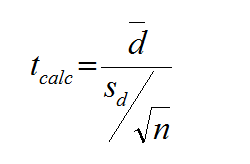# Tag Archives | hypothesis test## Paired t Test with SigmaXL

Paired t Test The third type of a Two Sample t-Test is the Paired t Test.  This test is used when the two populations are dependent of each other, so each data point from one distribution corresponds to a data point in the other distribution. When using a paired t test, the test statistic is calculated […]